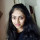Coffee Room
Discuss anything here - everything that you wish to discuss with fellow engineers.
12913 Members
Join this group to post and comment.# Solve : Triangle Problem :What Will Be The Answer To This Question ?

There is one question asked in Aptitude Test 1 in Aptitude section on CE.

Could anyone please solve and let us know the correct answer.

## There is triangle ABC with AB = AC and angle ABC = 40. If O is the center of the circle inscribed in triangle ABC, then find angle OAB.

Answer Options: 20, 50 80, 100Kaustubh Katdare • Oct 4, 2018

Okay, the question was wrong - and it's been fixed now. The question is -  Find the angle OABKnowns:

O is the center of the isosceles triangle with sides AB and AC equal,

Angle ABC = 40

Consequently, Angle ACB = 40

Unknowns:

Angle OAB = 1/2 of angle BAC

Angle BAC = 180 - sum of angles ABC and ACB (since, sum of angles of a triangle = 180

= 180 -  (40+40)

= 100

Finally,

Angle OAB = 1/2 of angle BAC

= 1/2 of 100

= 50.

The answer is 50.